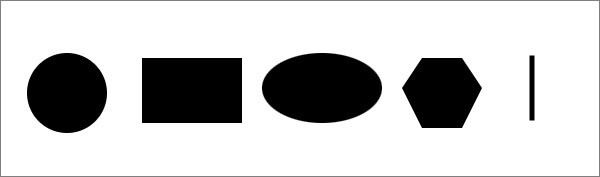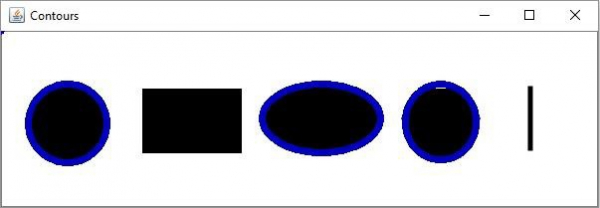# How to fit ellipses around possible objects in an image using OpenCV Java?

You can fit an ellipse over a shape using the fitEllipse() method of the org.opencv.imgproc.Imgproc class. This method accepts an object of MatOfPoint2f class, calculates the ellipse that would fit the given set of points and returns a RotatedRect object.

Using this you can draw ellipses around the possible objects in an image. To do so,

• Read an image using the imread() method of the Imgproc class.

• Convert it into a grayscale image using the cvtColor() method of the Imgproc class.

• Convert the gray image to binary using the threshold() method of the Imgproc class.

• Find the contours in the image using the findContours() method of the Imgproc class.

• Now, get the RotatedRec objects for the possible contours bypassing each contour value as MatOfPoint2f to the fitEllipse() method.

• Finally, draw ellipses around the possible shapes using the ellipse() method.

Note − To fit the ellipse the object should contain at least five points.

## Example

import java.util.ArrayList;
import java.util.List;
import org.opencv.core.Core;
import org.opencv.core.Mat;
import org.opencv.core.MatOfPoint;
import org.opencv.core.MatOfPoint2f;
import org.opencv.core.RotatedRect;
import org.opencv.core.Scalar;
import org.opencv.highgui.HighGui;
import org.opencv.imgcodecs.Imgcodecs;
import org.opencv.imgproc.Imgproc;
public class FitEllipseExample {
public static void main(String args[]) throws Exception {
//Reading the contents of the image
String file ="D:\Images\javafx_graphical.jpg";
//Converting the source image to binary
Mat gray = new Mat(src.rows(), src.cols(), src.type());
Imgproc.cvtColor(src, gray, Imgproc.COLOR_BGR2GRAY);
Mat binary = new Mat(src.rows(), src.cols(), src.type(), new Scalar(0));
Imgproc.threshold(gray, binary, 100, 255, Imgproc.THRESH_BINARY_INV);
//Finding Contours
List<MatOfPoint> contours = new ArrayList<>();
Mat hierarchey = new Mat();
Imgproc.findContours(binary, contours, hierarchey, Imgproc.RETR_TREE,
Imgproc.CHAIN_APPROX_SIMPLE);
//Empty rectangle
RotatedRect[] rec = new RotatedRect[contours.size()];
for (int i = 0; i < contours.size(); i++) {
rec[i] = new RotatedRect();
if (contours.get(i).rows() > 5) {
rec[i] = Imgproc.fitEllipse(new MatOfPoint2f(contours.get(i).toArray()));
}
Scalar color_elli = new Scalar(190, 0, 0);
Imgproc.ellipse(src, rec[i], color_elli, 5);
}
HighGui.imshow("Contours operation", src);
HighGui.waitKey();
}
}

## Input Image## Output